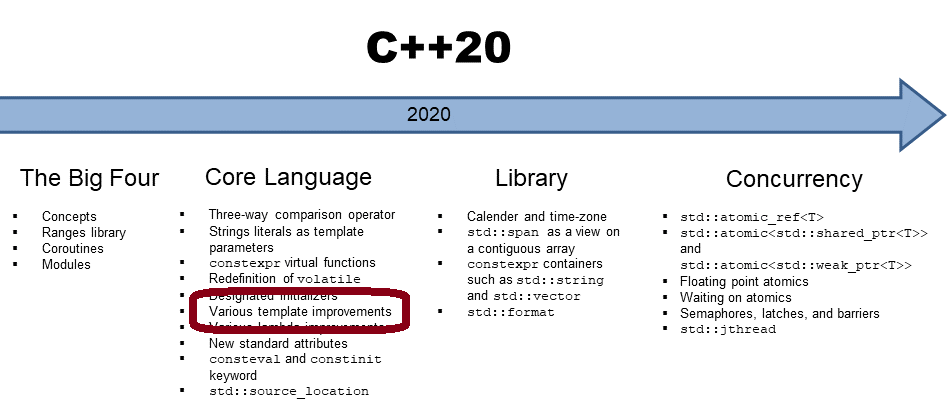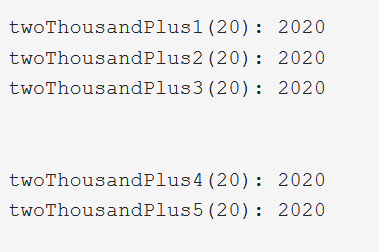# More and More Utilities in C++20

Today, I present a few utilities for calculating the midpoint of two values, checking if a `std::string` starts or ends with a substring, and creating callables with` std::bind_front`. These little utilities may not seem so minor when you need them.## Midpoint and Linear Interpolation

• `std::midpoint(a, b)` calculates the midpoint` (a + (b - a) / 2)` of the integers, floating points, or pointers. If a and b are pointers, they must point to the same array object.
• `std::lerp(a, b, t)` calculates the linear interpolation (a + t( b – a)). When t is outside the range [0, 1], it calculates the linear extrapolation.

The following program applies both functions.

```// midpointLerp.cpp

#include <cmath>     // std::lerp
#include <numeric>   // std::midpoint
#include <iostream>

int main() {

std::cout << std::endl;

std::cout << "std::midpoint(10, 20): " << std::midpoint(10, 20) << std::endl;

std::cout << std::endl;

for (auto v: {0.0, 0.1, 0.2, 0.3, 0.4, 0.5, 0.6, 0.7, 0.8, 0.9, 1.0}) {
std::cout << "std::lerp(10, 20, " << v << "): " << std::lerp(10, 20, v) << std::endl;
}

}
```

##Modernes C++ Mentoring

Be part of my mentoring programs:

• "Fundamentals for C++ Professionals" (open)
• "Design Patterns and Architectural Patterns with C++" (open)
• "C++20: Get the Details" (reopens December 2023)
• Do you want to stay informed about my mentoring programs: Subscribe via E-Mail.

The output of the program should be self-explanatory. If not, try it out on Compiler Explorer.C++20 has convenience functions for creating arrays.

## Creating Arrays and

With `std::to_array, `and `std::make_shared,` C++20 offers new  ways to create a `std::array` or `std::shared_ptr` from C-arrays.

### `std::to_array`

Thanks to` std::to_array`, creating a `std::array` from a C-array is a straightforward job.

```// toArray.cpp

#include <type_traits>
#include <utility>
#include <array>

int main(){

auto arr1 = std::to_array("C-String Literal");
static_assert(arr1.size() == 17);                  // (1)

auto arr2 = std::to_array({ 0, 2, 1, 3 });         // (2)
static_assert(std::is_same<decltype(arr2), std::array<int, 4>>::value);

auto arr3 = std::to_array<long>({ 0, 1, 3 });      // (3)
static_assert(std::is_same<decltype(arr3), std::array<long, 3>>::value);

auto arr4 = std::to_array<std::pair<int, float>>( { { 3, .0f }, { 4, .1f }, { 4, .1e23f } });
static_assert(arr4.size() == 3);                  // (4)
static_assert(std::is_same<decltype(arr4), std::array<std::pair<int, float>, 3>>::value);

}
```

The lines (1), (2), (3), and (3) assert that the created std::array has the expected type and size.

Per design, a `std::array` is as cheap and as fast as a C-array. If you want to know more about` std::array`, and why you should not use a C-array, read my post “std::array – Dynamic Memory, no Thanks“.

Additionally, a` std::array` knows its size and supports the typical interface of each container of the Standard Template Library, such as `std::vector`.

So far, all MSVC, Clang, GCC compilers support this convenient way to create a std::array. This observation does not hold for the next feature.

### `Create a std::shared_ptr of C-arrays`

Since C++11, C++ has the factory function `std::make_shared` to create a `std::shared_ptr`. Since C++20, `std::make_shared` also supports the creation of `std::shared_ptr` of C-arrays.

```auto s1 = std::make_shared<double[]>(1024);
auto s2 = std::make_shared<double[]>(1024, 1.0);
```

`s1` is a `std::shared_ptr `of a C-array. All members are default initialized. s2 is a `std::shared_ptr` of a C-array. Each element is initialized to `1.0.`

In contrast, the new two new member functions of `std::string` are already available with a brand-new MSVC, Clang, or GCC compiler.

## Check if a String starts with a Prefix or ends with a Suffix

`std::string` get a new member functions `starts_with` and` ends_with` which checks if a `std::string` start or ends with a specified substring

```// stringStartsWithEndsWith.cpp

#include <iostream>
#include <string_view>
#include <string>

template <typename PrefixType>
void startsWith(const std::string& str, PrefixType prefix) {
std::cout << "            starts with " << prefix << ": "
<< str.starts_with(prefix) << '\n';    // (1)
}

template <typename SuffixType>
void endsWith(const std::string& str, SuffixType suffix) {
std::cout << "            ends with " << suffix << ": "
<< str.ends_with(suffix) << '\n';
}

int main() {

std::cout << std::endl;

std::cout << std::boolalpha;

std::string helloWorld("Hello World");

std::cout << helloWorld << std::endl;

startsWith(helloWorld, helloWorld);                 // (2)

startsWith(helloWorld, std::string_view("Hello"));  // (3)

startsWith(helloWorld, 'H');                        // (4)

std::cout << "\n\n";

std::cout << helloWorld << std::endl;

endsWith(helloWorld, helloWorld);

endsWith(helloWorld, std::string_view("World"));

endsWith(helloWorld, 'd');

}
```

Both member functions `starts_with` end `ends_with` are predicates. This means they return a boolean. You can invoke the member function` starts_with` (line 1) with a` std::string` (line 2), a `std::string_view` (line 3), and a `char` (line 4).The following utility function in C++20 may wonder you.

## std::bind_front

`std::bind_front (Func&& func, Args&& ... args`) creates a callable wrapper for a callable` func. std::bind_front` that can have an arbitrary number of arguments and binds its arguments to the front.

Now, to the part which may wonder you. Since C++11, we have` std::bind` and lambda expression. To be pedantic` std::bind` is available since Technical Report 1 (TR1). Both can be used as a replacement of` std::bind_front`. Furthermore, `std::bind_front` seems like the minor sister of `std::bind,` because `std::bind` only supports the rearranging of arguments. Of course, there is a reason in the future to use `std::bind_front:` `std::bind_front` propagates exception specification of the underlying call operator.

The following program exemplifies that you can replace `std::bind_front `with` ``std::bind,` or lambda expressions.

```// bindFront.cpp

#include <functional>
#include <iostream>

int plusFunction(int a, int b) {
return a + b;
}

auto plusLambda = [](int a, int b) {
return a + b;
};

int main() {

std::cout << std::endl;

auto twoThousandPlus1 = std::bind_front(plusFunction, 2000);         // (1)
std::cout << "twoThousandPlus1(20): " << twoThousandPlus1(20) << std::endl;

auto twoThousandPlus2 = std::bind_front(plusLambda, 2000);           // (2)
std::cout << "twoThousandPlus2(20): " << twoThousandPlus2(20) << std::endl;

auto twoThousandPlus3 = std::bind_front(std::plus<int>(), 2000);     // (3)
std::cout << "twoThousandPlus3(20): " << twoThousandPlus3(20) << std::endl;

std::cout << "\n\n";

using namespace std::placeholders;

auto twoThousandPlus4 = std::bind(plusFunction, 2000, _1);           // (4)
std::cout << "twoThousandPlus4(20): " << twoThousandPlus4(20) << std::endl;

auto twoThousandPlus5 =  [](int b) { return plusLambda(2000, b); };  // (5)
std::cout << "twoThousandPlus5(20): " << twoThousandPlus5(20) << std::endl;

std::cout << std::endl;

}
```

Each call (lines 1 – 5) gets a callable taking two arguments and returns a callable taking only one argument because the first argument is bound to `2000`. The callable is a function (1), a lambda expression (2), and a predefined function object (line 3). `_1` is a so-called placeholder (line 4) and stands for the missing argument. With lambda expression (line 5), you can directly apply one argument and provide an argument `b` for the missing parameter. From the readability perspective,` std::bind_front` is easier to read than `std::bind`, or the lambda expression.If you want to play with the example, use Compiler Explorer.

## What’s next?

In my next post to C++20, I present the extensions of the chrono library: time of day, a calendar, and time zones.

Thanks a lot to my Patreon Supporters: Matt Braun, Roman Postanciuc, Tobias Zindl, G Prvulovic, Reinhold Dröge, Abernitzke, Frank Grimm, Sakib, Broeserl, António Pina, Sergey Agafyin, Андрей Бурмистров, Jake, GS, Lawton Shoemake, Jozo Leko, John Breland, Venkat Nandam, Jose Francisco, Douglas Tinkham, Kuchlong Kuchlong, Robert Blanch, Truels Wissneth, Kris Kafka, Mario Luoni, Friedrich Huber, lennonli, Pramod Tikare Muralidhara, Peter Ware, Daniel Hufschläger, Alessandro Pezzato, Bob Perry, Satish Vangipuram, Andi Ireland, Richard Ohnemus, Michael Dunsky, Leo Goodstadt, John Wiederhirn, Yacob Cohen-Arazi, Florian Tischler, Robin Furness, Michael Young, Holger Detering, Bernd Mühlhaus, Matthieu Bolt, Stephen Kelley, Kyle Dean, Tusar Palauri, Dmitry Farberov, Juan Dent, George Liao, Daniel Ceperley, Jon T Hess, Stephen Totten, Wolfgang Fütterer, Matthias Grün, Phillip Diekmann, Ben Atakora, Ann Shatoff, Rob North, and Bhavith C Achar.

Thanks, in particular, to Jon Hess, Lakshman, Christian Wittenhorst, Sherhy Pyton, Dendi Suhubdy, Sudhakar Belagurusamy, Richard Sargeant, Rusty Fleming, John Nebel, Mipko, Alicja Kaminska, Slavko Radman, and David Poole.

 My special thanks to EmbarcaderoMy special thanks to PVS-StudioMy special thanks to Tipi.buildMy special thanks to Take Up Code## Seminars

I’m happy to give online seminars or face-to-face seminars worldwide. Please call me if you have any questions.

### Standard Seminars (English/German)

Here is a compilation of my standard seminars. These seminars are only meant to give you a first orientation.

• C++ – The Core Language
• C++ – The Standard Library
• C++ – Compact
• C++11 and C++14
• Concurrency with Modern C++
• Design Pattern and Architectural Pattern with C++
• Embedded Programming with Modern C++
• Generic Programming (Templates) with C++

#### New

• Clean Code with Modern C++
• C++20

### Contact Me

Modernes C++ Mentoring,0 replies